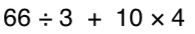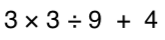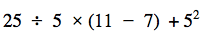Algebraic Equations
Exponents
Order of Operations
100

Solve for X:

X + 8 = 25

x=17

100

When you see the following expression, what is the big number called and what is the little number on top of it called?

24

100

Simplify the following expression:100
Simplify:

2x + 8x=

10x

200

Solve for X:

X-3=10

X=13

200

How can you rewrite 9in expanded form?

9 x 9

200

Simplify the following expression:200

Simplify:

4x + 2x -8x

-2x

300
Solve for X:

4x=28

X=7

300

How can you rewrite the following in exponential form?

7 x 7 x 7 x 7 x 7

75

300

3 + 42 * (3 + 2)

83

300
Simplify:

-3x + 2x +5 -2

-x + 3

400

Solve for X:X=40

400

What is the value of:

34

81

40045

400

Simplify:

-4x +2x -6 +3

-2x-3

500

Solve for X:

2x + 5 = 17

X=6

500

What is the value of:

23 + 32 - 42

50012

500

Simplify:

-2(x + 4) + 5x

-8 + 3x

Click to zoom